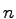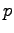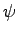## 1. 1. 4 Discretization

Discretization is required for obtaining an appropriate solution of a mathematical problem. It is used to transform the initially continuous problem which has an infinite number of degrees of freedom (e.g. eigenfunctions, Green's functions) into a discrete problem where the degree of freedom is inevitably limited. This limitation is necessary due to the finite nature of the subsequent calculation process.

Function spaces, required to form all possible solutions when applying arbitrary initial as well as boundary conditions, have an infinite dimension. Accordingly, the representation of a solution vector is not possible within the computer. The step of discretization is used to find a function space with a reasonable finite number of base functions, which comprises a proper approximation of the analytical solution.

In many cases base functions or shape functions with a local support are used. In this case the rather abstract task of finding shape functions can be reduced to the simpler task of finding a finite tesselation of the simulation domain.

At this point the physical origin of the mathematical formulation is neglected. The following steps do only rely on the posed mathematical model of the physical problem. This avoids post-implementation fittings which are not based on the continuous mathematical formulation but on the intuition of the engineer.

The following examples show alterations of mathematical and physical methods which are valid within the layer structure, because they origin from the mathematical formulation only.

Example 1: The use of a functional expression, which characterizes the physical behavior, for instance the mobility carrier of a material, is a purely physical adaption and results in changes of the posed differential equation and is a modeling approach.

Example 2: The Scharfetter Gummel Discretization  is a typical mathematical adaption of a discretization scheme with respect to the mathematical character of the functions,and. Even though the intentions initially did have physical nature, the method itself is formulated only by relying on the underlying differential equations. Further physical effects are not introduced.

All changes of the final discretization formulae due to physical reasons, which cannot be directly derived from the underlying physical model and its mathematical formulation are doubtful in their physical as well as mathematical meaning, because this is neither implied by a concise modeling approach, nor do such methods use a proper mathematical solution procedure. Of course, there is a certain chance to obtain acceptable results for special cases, but the overall credibility of such a system is corrupted due to the fact, that neither the mathematical methods nor the model can be benchmarked for validity and reproducibility of the results is not given.

It has to be stated that many approaches have combined the discretization (including assembly) of differential equations and the solution of the resulting algebraic problems. Even though at first glance it seems to be straight-forward to combine these two steps, one must remark that the implementation of all combinations, for instance for testing the optimum method, results in a growing implementation overhead.

Michael 2008-01-16